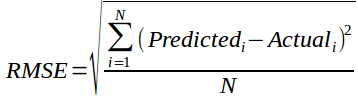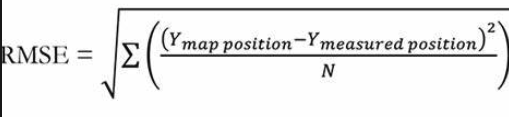# What is Root Mean Squared Error (RMSE)?

RMSE is the most popular evaluation metric used in regression problems. It follows an assumption that error are unbiased and follow a normal distribution. Here are the key points to consider on RMSE:

1. The power of ‘square root’ empowers this metric to show large number deviations.
2. The ‘squared’ nature of this metric helps to deliver more robust results which prevents cancelling the positive and negative error values. In other words, this metric aptly displays the plausible magnitude of error term.
3. It avoids the use of absolute error values which is highly undesirable in mathematical calculations.
4. When we have more samples, reconstructing the error distribution using RMSE is considered to be more reliable.
5. RMSE is highly affected by outlier values. Hence, make sure you’ve removed outliers from your data set prior to using this metric.
6. As compared to mean absolute error, RMSE gives higher weightage and punishes large errors.

RMSE metric is given by:where, N is Total Number of Observations.

The following steps are the procedure for calculating RMSE-• Calculate the square difference between all predicted data points and actual data points
• Calculate the mean of all these squared differences.
• Calculate the square root of the mean.

This is called as Root mean square error.

It is considered as the potential error which might happen during the prediction of a data point.

Let’s consider that you are predicting the mark of a student in the exam as 70 (out of 100) and let RMSE=12.

Then we can strongly assume that the actual mark of the student may come around [58, 82].

It indicates the power of prediction and possible change from the expectation.